Home > CCA2 > Chapter 11 > Lesson 11.2.2 > Problem11-57

11-57.
1. Sketch the graph of g(x) = (x − 2)2 − 1. 11-57 HW eTool (Desmos). Homework Help ✎

1. On the same set of axes (in a different color) sketch the graph of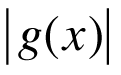, which is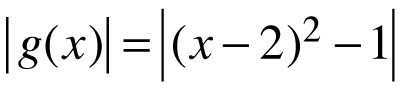.

2. On a new set of axes, sketch the graph of g(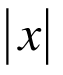), which is g() = (− 2)2 − 1.

3. Describe how each of the graphs in parts (a) and (b) are related to the original graph of g(x).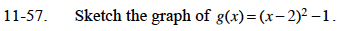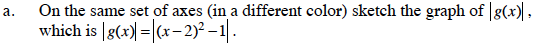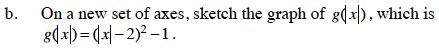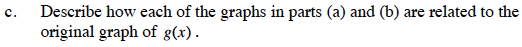Where are the graphs the same? Where are they different?

Use the eTool below to complete the parts of the problem.
Click the link at right for the full version of the eTool: CCA2 11-57 HW eTool.